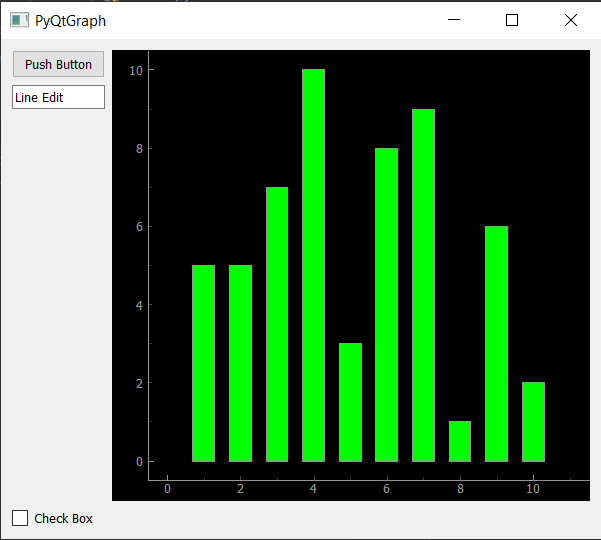# PyQtGraph – Adding Qt Widgets with the Bar Graph

• Last Updated : 30 Nov, 2021

In this article we will see how we can add Qt widgets with the bar graph in the PyQtGraph module. PyQtGraph is a graphics and user interface library for Python that provides functionality commonly required in designing and science applications. Its primary goals are to provide fast, interactive graphics for displaying data (plots, video, etc.) and second is to provide tools to aid in rapid application development (for example, property trees such as used in Qt Designer).A bar chart or bar graph is a chart or graph that presents categorical data with rectangular bars with heights or lengths proportional to the values that they represent. The bars can be plotted vertically or horizontally. A vertical bar chart is sometimes called a column chart. Qt have lots of multipurpose widgets which can be added with the bar graph so that it can become more interactive.
We can create a plot window and bar graph with the help of commands given below

```# creating a pyqtgraph plot window
window = pg.plot()

# creating a bar graph of green color
bargraph = pg.BarGraphItem(x=x, height=y1, width=0.6, brush='g')```

In order to do this we have to do the following
1. Import the QWidgets from the PyQt5
2. Import the pyqtgraph module
3. Create the main window class
4. Add various feature to the main window class
5. Create different types of QtWidgets
6. Create a plot window in which bar graph is added
7. Create a grid layout and add different widgets and plot window to it
8. Set the layout widget as the central widget of the window

Below is the implementation

## Python3

 `# importing Qt widgets``from` `PyQt5.QtWidgets ``import` `*` `import` `sys` `# importing pyqtgraph as pg``import` `pyqtgraph as pg`   `class` `Window(QMainWindow):` `    ``def` `__init__(``self``):``        ``super``().__init__()` `        ``# setting title``        ``self``.setWindowTitle(``"PyQtGraph"``)` `        ``# setting geometry``        ``self``.setGeometry(``100``, ``100``, ``600``, ``500``)` `        ``# calling method``        ``self``.UiComponents()` `        ``# showing all the widgets``        ``self``.show()` `    ``# method for components``    ``def` `UiComponents(``self``):` `        ``# creating a widget object``        ``widget ``=` `QWidget()` `        ``# creating a push button object``        ``btn ``=` `QPushButton(``'Push Button'``)` `        ``# creating a line edit widget``        ``text ``=` `QLineEdit(``"Line Edit"``)` `        ``# creating a check box widget``        ``check ``=` `QCheckBox(``"Check Box"``)` `        ``# creating a plot window``        ``plot ``=` `pg.plot()` `        ``# create list for y-axis``        ``y1 ``=` `[``5``, ``5``, ``7``, ``10``, ``3``, ``8``, ``9``, ``1``, ``6``, ``2``]` `        ``# create horizontal list i.e x-axis``        ``x ``=` `[``1``, ``2``, ``3``, ``4``, ``5``, ``6``, ``7``, ``8``, ``9``, ``10``]` `        ``# create pyqt5graph bar graph item``        ``# with width = 0.6``        ``# with bar colors = green``        ``bargraph ``=` `pg.BarGraphItem(x ``=` `x, height ``=` `y1, width ``=` `0.6``, brush ``=``'g'``)` `        ``# add item to plot window``        ``# adding bargraph item to the plot window``        ``plot.addItem(bargraph)` `        ``# Creating a grid layout``        ``layout ``=` `QGridLayout()` `        ``# setting this layout to the widget``        ``widget.setLayout(layout)` `        ``# adding widgets in the layout in their proper positions``        ``# button goes in upper-left``        ``layout.addWidget(btn, ``0``, ``0``)` `        ``# text edit goes in middle-left``        ``layout.addWidget(text, ``1``, ``0``)` `        ``# check box widget goes in bottom-left``        ``layout.addWidget(check, ``3``, ``0``)` `        ``# plot window goes on right side, spanning 3 rows``        ``layout.addWidget(plot, ``0``, ``1``, ``3``, ``1``)` `        ``# setting this widget as central widget of the main window``        ``self``.setCentralWidget(widget)`  `# create pyqt5 app``App ``=` `QApplication(sys.argv)` `# create the instance of our Window``window ``=` `Window()` `# start the app``sys.exit(App.``exec``())`

Output :My Personal Notes arrow_drop_up